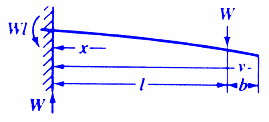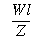Bending, Deflection and Stress Equations Calculator for

Beam Deflection and Stress Formula and Calculators

Structural Beam Deflection, Stress, Bending Equations and calculator for a Cantilevered Beam with Load at Location Between Support and Load.Stress at specific pointStress at the support (must be constant cross section)Maximum deflection at endWhere:

 E = Modulus of Elasticity psi (N/mm2) I = Moment of Inertia in4 (mm4) W = Load lbs (N) s = Stress at the cross-section being evaluated lbs/in2 (N/mm2) y = Deflection inches (mm)= Some distance as indicated inches (mm) x = Some distance as indicated inches (mm) v = Some distance as indicated inches (mm) b = Some distance as indicated inches (mm) Z = section modulus of the cross-section of the beam = I/z in3 (mm3) z = distance from neutral axis to extreme fiber (edge) inches (mm)
• Please note letter "" (lower case "L") is different than "I" (Moment of Inertia).
• Deflections apply only to constant cross sections along entire length.

References:

• Any Machinery's Handbook published since 1931 or,
• Machinery's Handbook, 21st Edition, Page 408 or,
• Machinery's Handbook, 23st Edition, Page 264 or,
• Machinery's Handbook, 27st Edition, Page 265Membership Register | LoginHomeEngineering Book StoreEngineering ForumExcel App. DownloadsOnline Books & ManualsEngineering NewsEngineering VideosEngineering CalculatorsEngineering ToolboxGD&T Training Geometric Dimensioning TolerancingDFM DFA TrainingTraining Online EngineeringAdvertising CenterCopyright Notice